# Right Angle - Definition with Examples

The Complete K-5 Math Learning Program Built for Your Child

• 30 Million Kids

Loved by kids and parent worldwide

• 50,000 Schools

Trusted by teachers across schools

• Comprehensive Curriculum

Aligned to Common Core

## Right Angle GamesRight Angle

Identify right angles in different two dimensional shapes. Remember, you can recognize right angles by looking at perpendicular lines.

Covers Common Core Curriculum 4.G.2

## What is Right Angle?

In geometry, when two rays meet each at a common point, they form an angle. The point of meeting of two rays is known as the vertex.

Angles are measured in degrees (symbol: ˚)Some common types of angles are acute angles, right angles, and obtuse angles.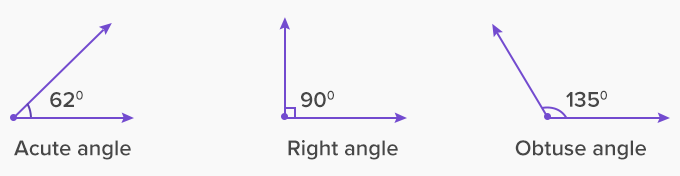Right angle

When two straight lines intersect each other at 90˚ or are perpendicular to each other at the intersection, they form the right angle. A right angle is represented by the symbol ∟.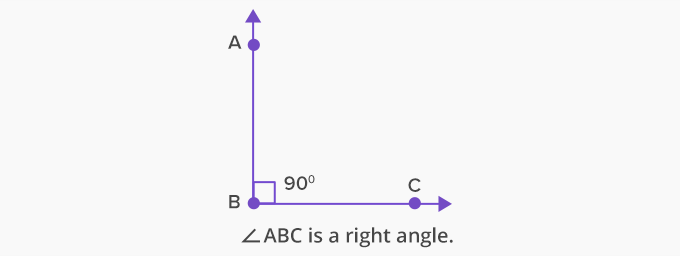The given image shows various formations of the right angle.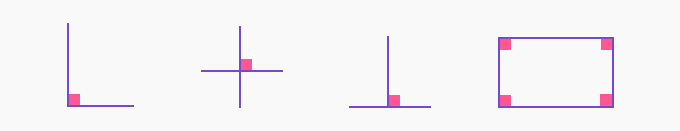We can find the right angles in shapes.

A square or rectangle has four corners with right angles.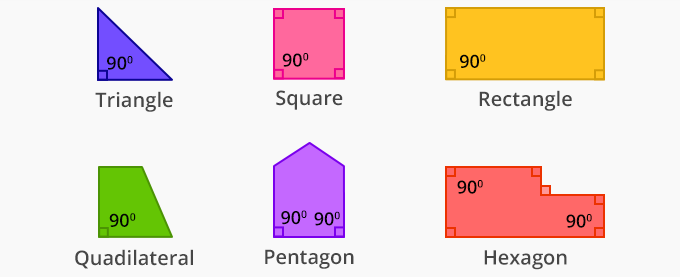Examples of right angles are all around us. We can see right angles in the corners of a room, book, cube, windows and at several other places.A vertical and a horizontal line make most common right angles. However, diagonal lines intersecting each other also form right angles. If you draw the diagonals of a square, a rhombus or a kite, the angle at the intersection is 90 degrees and is, therefore, a right angle.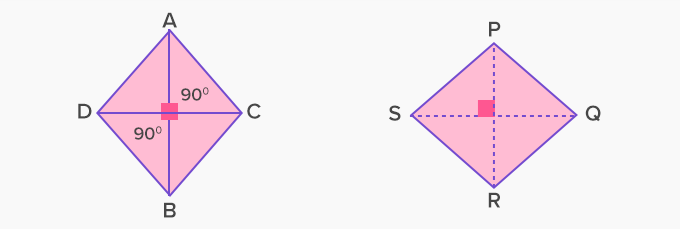Example of a rhombus and a kite with diagonals intersecting at a right angle.

How to draw a right angle using a protractor?

1. Start by drawing a horizontal line.2. Now place the protractor on the horizontal line.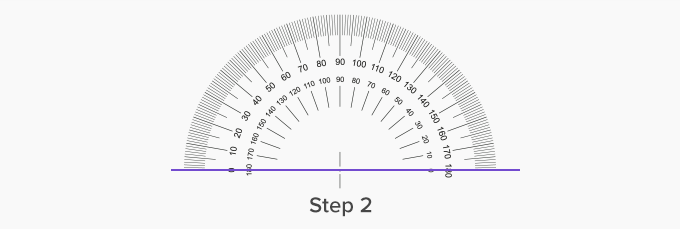3. Measure 90˚ and mark it with a point.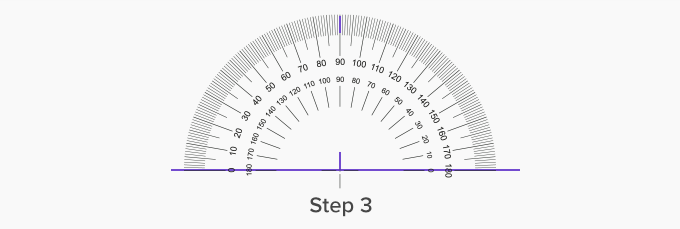4. Now using a scale, draw a straight line from this point to the horizontal line.Fun Facts  All right angles are the same. All right angles correspond to quarter of a complete turn.  All triangles with one angle right are called right-angled triangles.

Won Numerous Awards & Honors